# The TTEST Procedure

#### Paired Design

Define the following notation: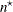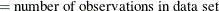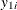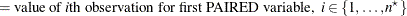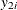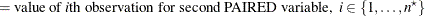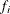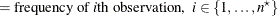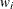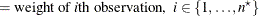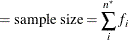##### Normal Difference (DIST=NORMAL TEST=DIFF)

The analysis is the same as the analysis for the one-sample design in the section Normal Data (DIST=NORMAL) based on the differences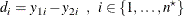##### Lognormal Ratio (DIST=LOGNORMAL TEST=RATIO)

The analysis is the same as the analysis for the one-sample design in the section Lognormal Data (DIST=LOGNORMAL) based on the ratios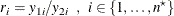##### Normal Ratio (DIST=NORMAL TEST=RATIO)

The hypothesis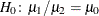, where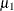and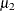are the means of the first and second PAIRED variables, respectively, can be rewritten as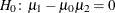. The t value and p-value are computed in the same way as in the one-sample design in the section Normal Data (DIST=NORMAL) based on the transformed values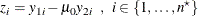Estimates and confidence limits are not computed for this situation.